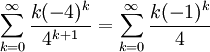# Power Series Convergence Example

Previous: Power Series Convergence

Next: Videos on Power Series

## Example

Determine the radius and interval of convergence of the infinite series## Complete Solution

### Step 1: Apply Ratio Test

The ratio test gives us:The ratio test tells us that the power series converges only whenor. Therefore, the radius of convergence is 4.

### Step 2: Test End Points of Interval to Find Interval of Convergence

The inequalitycan be written as -7 < x < 1. By the ratio test, we know that the series converges on this interval, but we don't know what happens at the points x = -7 and x = 1.

At x = -7, we have the infinite seriesThis series diverges by the test for divergence.

At x = 1, we have the infinite seriesThis series also diverges by the test for divergence.

Therefore, the interval of convergence is -7 < x < 1.

## Possible Challenges

### What Convergence Test Should Be Used?

For most problems, the ratio test can be used initially. If the ratio test yields an interval for the domain, we need to use other convergence tests to explore what the domain could be at the end points of the interval.

Previous: Power Series Convergence

Next: Videos on Power Series